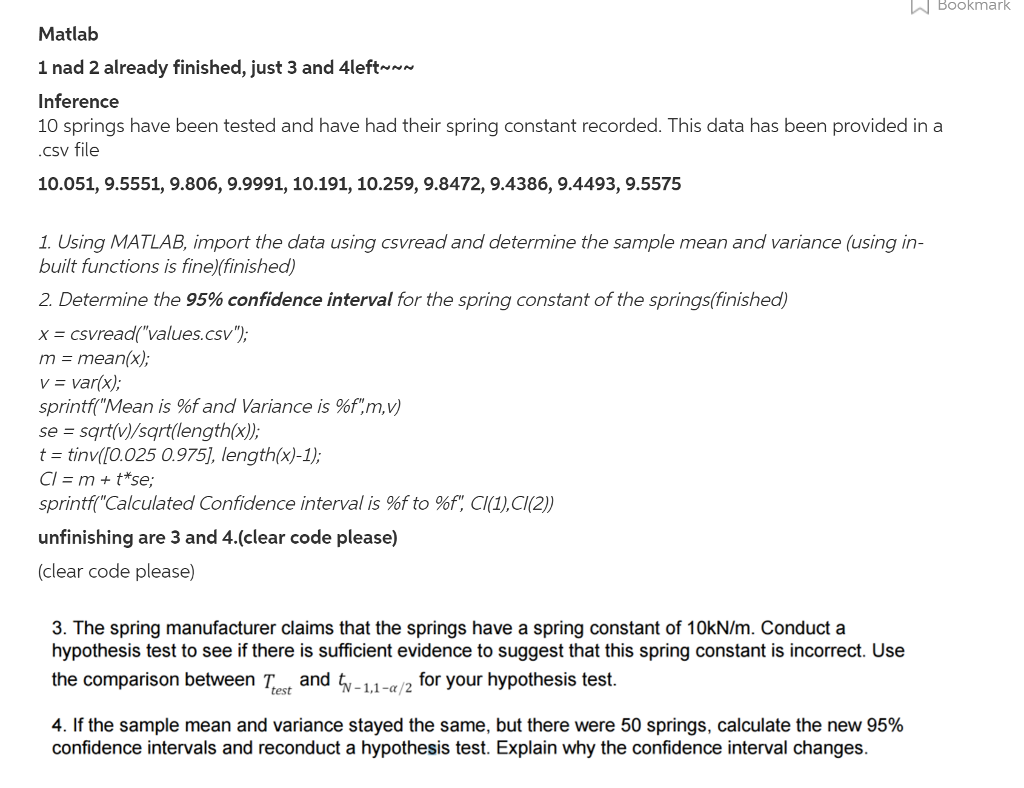# Question & Answer: 10 springs have been tested and have had their spring constant recorded. This data has been provided in a csv file…..

10 springs have been tested and have had their spring constant recorded. This data has been provided in a csv file 10.051, 9.5551, 9.806, 9.9991, 10.191, 10.259, 9.8472, 9.4386, 9.4493, 9.5575 1. Using MATLAB, import the data using csvread and determine the sample mean and variance using in built functions is fine)(finished) 2. Determine the 95% confidence interval for the spring constant of the springs finished) x = csvread(“values csv”) m = mean(x); v = var(x); sprintf (“Mean is %f and Variance is %f, m, v) se= squareroot (v)/ squareroot (length(x)); t = tinv ([0.025 0.975], length(x) – 1); Cl = m + t* se; sprintf “Calculated Confidence interval is %f to %f”, Cl(1), Cl(2)) unfinishing are 3 and 4.(clear code please) (clear code please) 3. The spring manufacturer claims that the springs have a spring constant of 10kN/m. Conduct a hypothesis test to see if there is sufficient evidence to suggest that this spring constant is incorrect. Use the comparison between test T_test and t_N, 1, 1 -alpha/2 for your hypothesis test. 4. If the sample mean and variance stayed the same, but there were 50 springs, calculate the new 95% confidence intervals and reconduct a hypothesis test. Explain why the confidence interval changes.Don't use plagiarized sources. Get Your Custom Essay on
Question & Answer: 10 springs have been tested and have had their spring constant recorded. This data has been provided in a csv file…..
GET AN ESSAY WRITTEN FOR YOU FROM AS LOW AS \$13/PAGE

3.

Hypotheses are:

Since population standard deviation is unknown and hypothesis test population mean so t test should be used. Here sample size is large so we can assume that sampling distribution of sample mean is normal.

So test statitics will be

Here test is two tailed and degree of freedom of the test is df=n-1=10-1=9. So for , critical value of the test are +/-2.2622. Rejection region:

If t< -2.2622 or t > 2.2622, we reject the null hypothesis.

Since test statistics value is not less than -2.2622 so we fail to reject the null hypothesis.

That is we cannot conclude that spring constant is different from 10 kN/m.

4:

Since there 50 data values in the sample so degree of freedom is df=50-1=49 and critical value of t for 95% confidence interval is +/- 2.0096. Therefore required confidence interval is

Therefore, a 95% confidence interval for the mean is (9.62138, 10.00938).

————————–

Hypotheses are:

Since population standard deviation is unknown and hypothesis test population mean so t test should be used. Here sample size is large so we can assume that sampling distribution of sample mean is normal.

So test statitics will be

Here test is two tailed and degree of freedom of the test is df=n-1=50-1=49. So for , critical value of the test are +/-2.0096. Rejection region:

If t< -2.0096 or t > 2.0096, we reject the null hypothesis.

Since test statistics value is less than -2.0096 so we reject the null hypothesis.

That is we can conclude that spring constant is different from 10 kN/m.